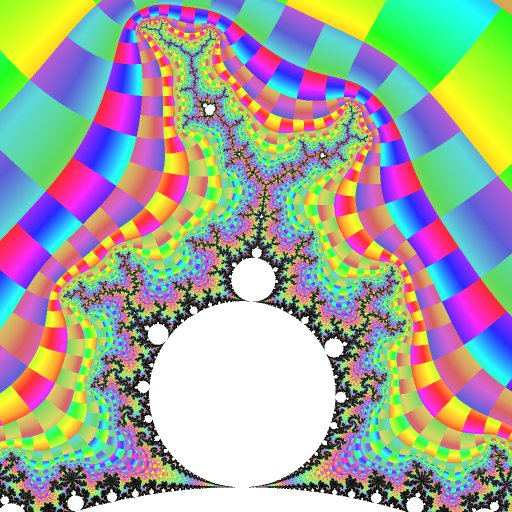mdbtxt1
mdbtxt2
Proceed to Safety

# R2.1/3

Robert P. Munafo, 2012 Mar 4.

R2.1/3 is the R2-name for a mu-unit of period 3. Its owner is the mu-atom R2.1/3a, located at approximately -0.122 + 0.745i.

R2.1/3a, R2.1/3, the radical R2F(1/3(*B)), the larger of the two primary filaments R2F(1/3B1), and its largest island R2F(1/3B1)S are each notable for being the largest non-symmetrical example of their class. Like all non-symmetrical features of the Mandelbrot set, R2.1/3a has an identical mirror-image, R2.2/3a, located at -0.122 - 0.745i.

R2.1/3a's bond point R2.b(1/3) is at precisely (-1 + 3 √3 i) / 8, as given by the general formulas for secondary continental mu-atoms (see that entry for more details). On either side of this bond point are the two cusps R2.C(1/3).

The centers of R2.1/3a and R2.2/3a are given by two of the solutions to the equation Z3 = 0, where Z3 is the 3rd lemniscate. The center of R2.1/3a is precisely:

(-√3i/2 - 1/2)/(9 (√23/(6 √3)-25/54)(1/3)) + (√23/(6 √3)-25/54)(1/3) (√3i/2-1/2) - 2/3

which is -0.12256116628477... + 0.74486176791682...i

As described in the Brown Method entry, there are also precise formulas for the boundaries of R2.1/3a and R2.2/3a.

See the mu-unit article for an image of R2.1/3 alone without the attached primary filaments.-0.1110 + 0.8843i @ 0.52 R2.1/3 -._/ ., ~/_c a/` ^,^ :` ''^e/_"- 1 ----> ..\$d/% _e .c ~ ' ')/ @`_, -`LaL ~,,e_/' ^` -"^C, -,e-"`"^'^ ^~\"*`"' ,:@\_ _ '-"(*~^ ._._T, .a/\$^.c _.a, ._ '^"^"@a(@^@)\e/%__ -\_- ., .a/F~ ^@F% ' a- ..m ,d_ee\$F '-\",__FY, ..FF` ._ /-_a@` "[^ ./ee.-a :d*v*"^^^``''*-L@.d)-. '\$*_,, :(@b**"LF^` '^TC`-m,_dF^"C:/ ^ mdL_,' ^"""*d\$d, ' :ae,-v/F \/F"-^ ._v~C_.L@ 'b*`.mva '^^ -YYe_ R2.1/3a ""bd"b, -mc\$[ .@"%^ "`"d-e .d\$mY^ . *"bm, ./)*` -_, ,/"_ .,._ _ ^"Ym,, _.m*^` _ .-m_, .._^@^ @""* aa@@d _/bb_mrae___d%me_, 2 ._am%%___aeam_:@d_ :@@@L_ '")v(d FTb@b._^mF'^Lr@`""`^^'`^ `'^'^'""`\$me@*"\F^, mmbaF@L '^'^^^^ ' ^ ^'^^ '^' `'^ -0.1110 + 0.8843i @ 0.52

Labeled features in the figure:

1. Technical: Period-4 mu-molecule

R2-Name: R2F(1/3B1)S

2. Technical: bond point

R2-Name: R2.b(1/3)

For more clarity on what is meant by the "mu-unit" R2.1/3, see the mu-unit heading.

 The roots of Z3 = 0 were evaluated in Maxima. Here is are the commands:

display2d:true; z2:c^2+c; z3:z2^2+c; r3:solve(z3=0,c); display2d:false; r3; float(r3); expand(%); float(%);

and here is what it produces:

Maxima 5.11.0 http://maxima.sourceforge.net Using Lisp SBCL 1.0.2 Distributed under the GNU Public License. See the file COPYING. Dedicated to the memory of William Schelter. This is a development version of Maxima. The function bug_report() provides bug reporting information.   (%i1) display2d:true;   (%o1) true   (%i2) z2:c^2+c; 2 (%o2) c + c   (%i3) z3:z2^2+c; 2 2 (%o3) (c + c) + c   (%i4) r3:solve(z3=0,c);   sqrt(3) %i 1 ---------- - - - 3/2 2 2 3 sqrt(23) 25 1/3 (%o4) [c = --------------------------- + (--------------- - --) - 3/2 2 54 3 sqrt(23) 25 1/3 9 (--------------- - --) 2 54 - 3/2 sqrt(3) %i 1 2 3 sqrt(23) 25 1/3 sqrt(3) %i 1 (- ---------- - -) - -, c = (--------------- - --) (---------- - -) 2 2 3 2 54 2 2 sqrt(3) %i 1 - ---------- - - 2 2 2 + --------------------------- - -, c = - 3/2 3 3 sqrt(23) 25 1/3 9 (--------------- - --) 2 54 - 3/2 3 sqrt(23) 25 1/3 1 2 (--------------- - --) + --------------------------- - -, c = 0] 2 54 - 3/2 3 3 sqrt(23) 25 1/3 9 (--------------- - --) 2 54   (%i5) display2d:false;   (%o5) false   (%i6) r3;   (%o6) [c = (sqrt(3)*%i/2-1/2)/(9*(3^-(3/2)*sqrt(23)/2-25/54)^(1/3)) +(3^-(3/2)*sqrt(23)/2-25/54)^(1/3)*(-sqrt(3)*%i/2-1/2)-2/3, c = (3^-(3/2)*sqrt(23)/2-25/54)^(1/3)*(sqrt(3)*%i/2-1/2) +(-sqrt(3)*%i/2-1/2)/(9*(3^-(3/2)*sqrt(23)/2-25/54)^(1/3))-2/3, c = (3^-(3/2)*sqrt(23)/2-25/54)^(1/3) +1/(9*(3^-(3/2)*sqrt(23)/2-25/54)^(1/3))-2/3,c = 0]   (%i7) float(r3);   (%o7) [c = -.05555555555555555*140175^(1/3)*(.8660254037844386*%i-0.5) /26^(1/3) -2.0*26^(1/3)*(-.8660254037844386*%i-0.5)/140175^(1/3) -.6666666666666666, c = -2.0*26^(1/3)*(.8660254037844386*%i-0.5)/140175^(1/3) -.05555555555555555*140175^(1/3)*(-.8660254037844386*%i-0.5)/26^(1/3) -.6666666666666666, c = -.05555555555555555*140175^(1/3)/26^(1/3) -2.0*26^(1/3)/140175^(1/3)-.6666666666666666,c = 0.0]   (%i8) expand(%);   (%o8) [c = -.04811252243246881*140175^(1/3)*%i/26^(1/3) +1.732050807568877*26^(1/3)*%i/140175^(1/3) +.02777777777777778*140175^(1/3)/26^(1/3)+1.0*26^(1/3)/140175^(1/3) -.6666666666666666, c = .04811252243246881*140175^(1/3)*%i/26^(1/3) -1.732050807568877*26^(1/3)*%i/140175^(1/3) +.02777777777777778*140175^(1/3)/26^(1/3)+1.0*26^(1/3)/140175^(1/3) -.6666666666666666, c = -.05555555555555555*140175^(1/3)/26^(1/3) -2.0*26^(1/3)/140175^(1/3)-.6666666666666666,c = 0.0]   (%i9) float(%);   (%o9) [c = -.7448617679168161*%i-.1225611662847714, c = .7448617679168161*%i-.1225611662847714,c = -1.754877667430457, c = 0.0]   (%i10) quit();

revisions: 20030922 oldest on record; 20100911 replace sqrt(3) with √3, etc.; 20100918 add image and more links; 20120304 link to R2.C(1/3)

From the Mandelbrot Set Glossary and Encyclopedia, by Robert Munafo, (c) 1987-2023.     Mu-ency index

This page was written in the "embarrassingly readable" markup language RHTF, and was last updated on 2012 Mar 05.s.27